# Data Representation in Computer Memory [Dev Concepts #33]

In this article of the series Dev Concepts, we take a look at the binary representation of integers, floating-point numbers, text, and unicode.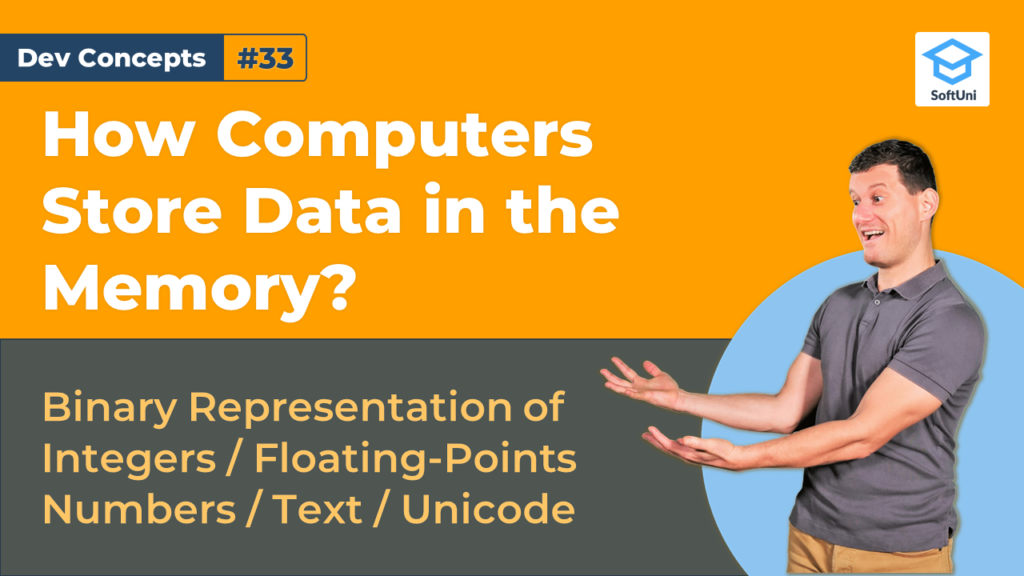In this lesson, we will talk about storing data in the computer memory. By the end of this article, you will know how to work with binary representation of integers, floating-point numbers, text, and Unicode.

Integer numbers are represented in the computer memory, as a sequence of bits: 8-bits, 16-bits, 24-bits, 32-bits, 64-bits, and others, but always a multiple of 8 (one byte). They can be signed or unsigned and depending on this, hold a positive, or negative value. Some values in the real world can only be positive – the number of students enrolled in a class. There can be also negative values in the real world such as daily temperature.

Positive 8-bit integers have a leading 0, followed by 7 other bits. Their format matches the pattern “0XXXXXXX” (positive sign + 7 significant bits). Their value is the decimal value of their significant bits (the last 7 bits).

Negative 8-bit integers have a leading one, followed by 7 other bits. Their format matches the pattern “1YYYYYYY” (negative sign + 7 significant bits). Their value is -128 (which is minus 2 to the power of 7) plus the decimal value of their significant bits.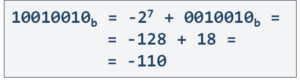Example of signed 8-bit binary integer

The table below summarizes the ranges of the integer data types in most popular programming languages, which follow the underlying number representations that we discussed in this lesson. Most programming languages also have 64-bit signed and unsigned integers, which behave just like the other integer types but have significantly larger ranges.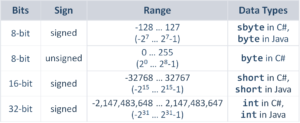• The 8-bit signed integers have a range from -128 to 127. This is the sbyte type in C# and the byte type in Java.
• The 8-bit unsigned integers have a range from 0 to 255. This is the byte type in C#.
• The 16-bit signed integers have a range from -32768 to 32767. This is the short type in Java, C#.
• The 16-bit unsigned integers have a range from 0 to 65536. This is the ushort type in C#.
• The 32-bit signed integers have a range from -231231-1 (which is from minus 2 billion to 2 billion roughly).  This is the int type in C#, Java, and most other languages. This 32-bit signed integer data type is the most often used in computer programming. Most developers write “int” when they need just a number, without worrying about the range of its possible values because the range of “int” is large enough for most use cases.

### Representing Text

Computers represent text characters as unsigned integer numbers, which means that letters are sequences of bits, just like numbers.

The ASCII standard represents text characters as 8-bit integers. It is one of the oldest standards in the computer industry, which defines mappings between letters and unsigned integers. It simply assigns a unique number for each letter and thus allows letters to be encoded as numbers.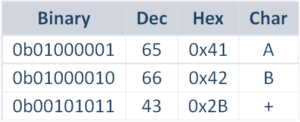For example, the letter “A” has ASCII code 65. The letter “B” has ASCII code 66. The “plus sign” has ASCII code 43. The hex and binary values are also shown and are useful in some situations.

### Representing Unicode Text

The Unicode standard represents more than 100,000 text characters as 16-bit integers. Unlike ASCII it uses more bits per character and therefore it can represent texts in many languages and alphabets, like Latin, Cyrillic, Arabic, Chinese, Greek, Korean, Japanese, and many others.

Here are a few examples of Unicode characters: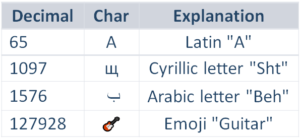• The Latin letter “A” has Unicode number 65.
• The Cyrillic letter “sht” has Unicode number 1097.
• The Arabic letter “beh” has Unicode number 1576.
• The “guitar” emoji symbol has Unicode number 127928.

In any programming language, we either declare data type before using a variable, or the language automatically assigns a specific data type. In this lesson, we have learned how computers store integer numbers, floating-point numbers, text, and other data. These concepts shouldn’t be taken lightly, and be careful with them!

## Lesson Topics

In this tutorial we cover the following topics:
• Representation of Data

• Representing Integers in Memory

• Representation of Signed Integers

• Largest and Smallest Signed Integers

• Integers and Their Ranges in Programming

• Representing Real Numbers

• Storing Floating-Point Numbers

• Representing Text and Unicode Text

• Sequences of Characters